Thermodynamics and Propulsion

# 10.1 An Expression of Newton's 2nd Law (e.g.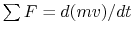)

Consider two coordinate systems:

1. Inertial (labeled with subscriptin Figure 10.1),
2. Fixed to vehicle (labeled with subscript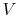in Figure 10.1)
• Moves with velocity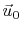relative to the inertial coordinate system.
• All velocities relative to the vehicle-fixed coordinate frame are denoted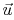.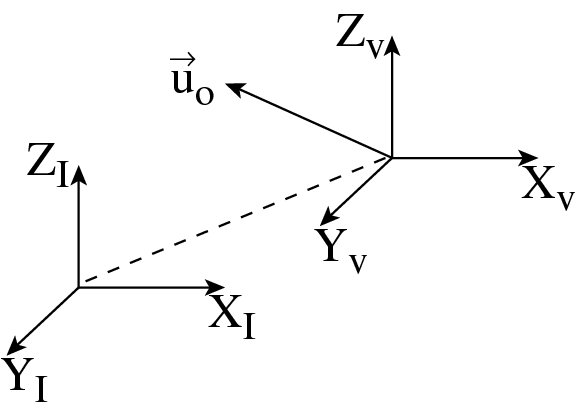Newton's second law for a control volume of fixed mass can be written as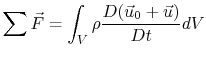or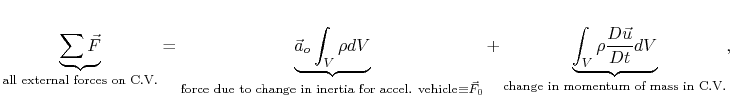where the external forces acting on the control volume may be pressures forces, shear forces (skin friction), and body forces.

To explain the above equation further, consider Figure 10.2.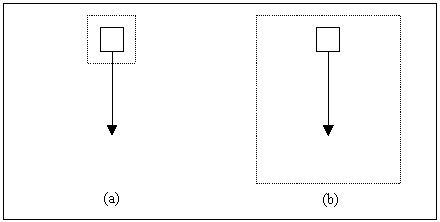The falling block labeled (a) has a control volume fixed to it. In this case, the first term of the above equation is nonzero since the control volume is accelerating relative to an inertial reference frame. The second term is zero because the block is not accelerating relative to a coordinate system fixed to the control volume. The opposite is true for the falling block labeled (b), which is falling within a fixed control volume. The first term of the above equation is zero in this case because the control volume is not accelerating relative to an inertial reference frame. The second term is nonzero because the block is moving to a coordinate system fixed to the control volume. The mathematical result of both cases is as follows: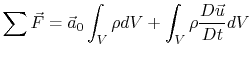1.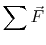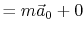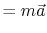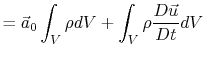2.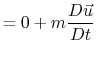As expected, the result is the same for both. The integral momentum equation reduces to a familiar form,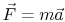. To continue, the integral momentum equation can be rewritten as follows,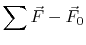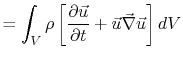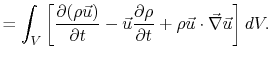From conservation of mass,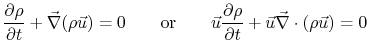so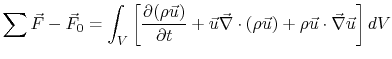Note that this is a vector equation. Considering only the components in the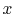-direction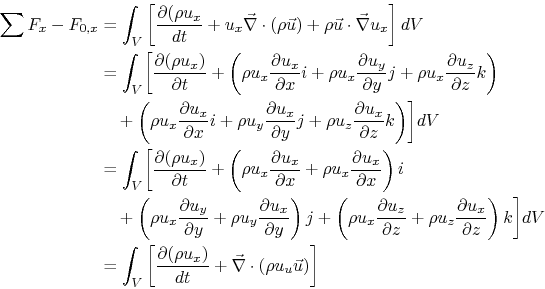Then, by the divergence theorem,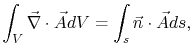where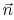is outward unit normal vector. We thus havewhere again the forces acting on the control volume may be composed of pressure forces, body forces, and skin friction.

For steady flow, with no acceleration of the vehicle then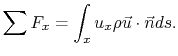This is the form we will use most frequently in this class.

UnifiedTP